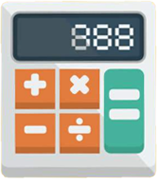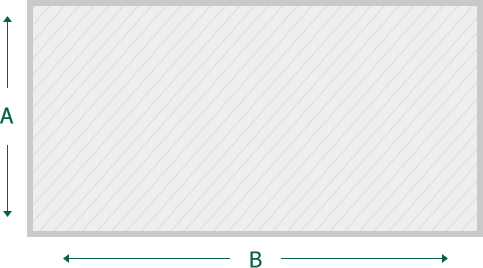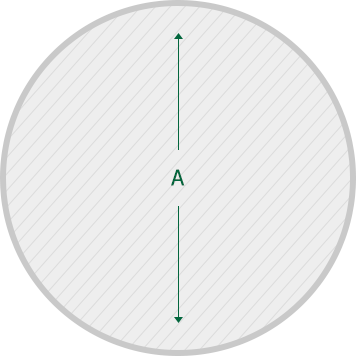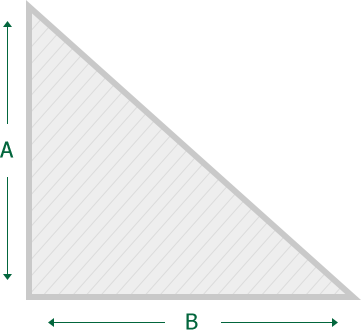Use our materials calculator to estimate how much material you will need for your project. See how much gravel you need for your driveway or sidewalk or pathways, play-sand for your sand box, topsoil for your lawn or yard, soil-mixes for your raised beds or mulch for your flower beds. Simply select the approximate shape of the area, the material type and enter the dimensions.Calculation Tips

Armour Stone Calculation

Length X Height X Depth = _______/12 is the amount of rock needed in tons for retaining walls.

Wall is 50 feet long stacked two high (18" rock) = 36 inches at a depth of 24 inches, then you will need: 25 tons of rock (50X3X2/12=25 tons).

Soil and Mulch Calculation

Length X Height X Depth = _______/27 is the amount of yards of product you need for you project.

Aggregates Calculation for Compacted Material

1 ton of compacted aggregate will cover:

1" thick = 200 square feet 2" thick = 100 square feet
3" thick = 75 square feet 4" thick = 50 square feet
5" thick = 38 square feet 6" thick = 25 square feet

2" thick = 100 square feet
4" thick = 50 square feet
6" thick = 25 square feet